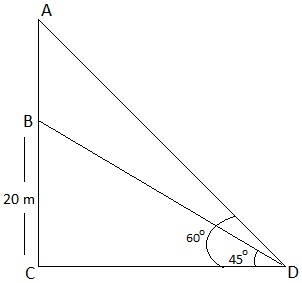Guru

# From a point on the ground, the angles of elevation of the bottom and the top of a transmission tower fixed at the top of a 20 m high building are 45° and 60° respectively. Find the height of the tower. Q.7

• 0

Sir please give me the best solution for the question for class 10th ncert book of exercise 9.1 of applications of Trigonometry chapter of question no.7, what is the simplest way for solving this problem From a point on the ground, the angles of elevation of the bottom and the top of a transmission tower fixed at the top of a 20 m high building are 45° and 60° respectively. Find the height of the tower.

Share

1. Let BC be the 20 m high building.

D is the point on the ground from where the elevation is taken.

Height of transmission tower = AB = AC – BCTo Find: AB, Height of the tower

From figure, In right ΔBCD,

tan 45° = BC/CD

1 = 20/CD

CD = 20

Again,

In right ΔACD,

tan 60° = AC/CD

√3 = AC/20

AC = 20√3

Now, AB = AC – BC = (20√3-20) = 20(√3-1)

Height of transmission tower = 20(√3 – 1) m.

• 0